# 10 Best Challenging Math Puzzles For Genius

Here you will get 10 best challenging math puzzles for genius. There are some logical reasoning mathematical puzzles that will help you to improve logical thinking. Mathematics helps us to solve our daily life problems. Our day will not pass without solving many of the mathematics problems.

1. Viral Math Puzzle that Stumps on the Internet

1+4=5, 2+5=12, 3+6=21, 8+11=?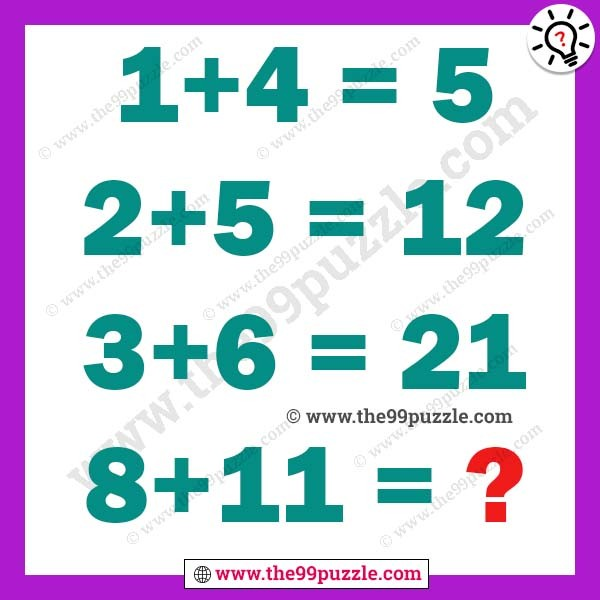2. Logic Math Brain Teaser For Genius With Answer

1+1+1+1+1

1+1+1+1+1

1+1×0+1=?3. Only genius can solve math logic

7×7=12, 5×5=8, 3×3=4, 2×2=2, 6×6=?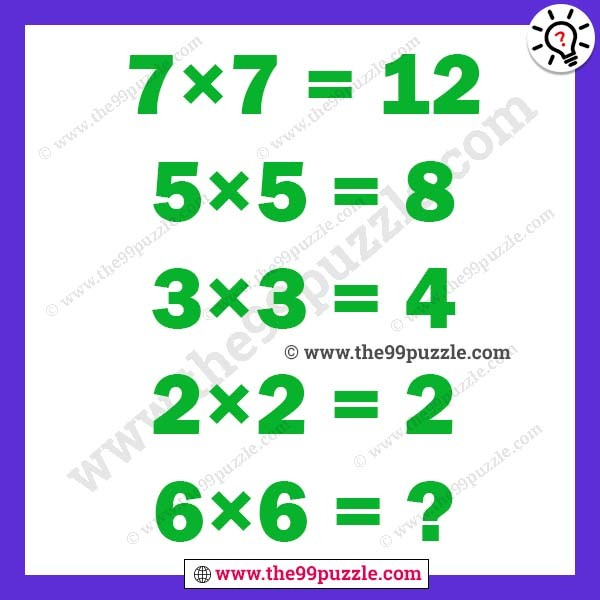4. Maths Logic Problem For Adults

3+3×4=28, 4+4×6=54, 5+5×7=77, 6+6×9=?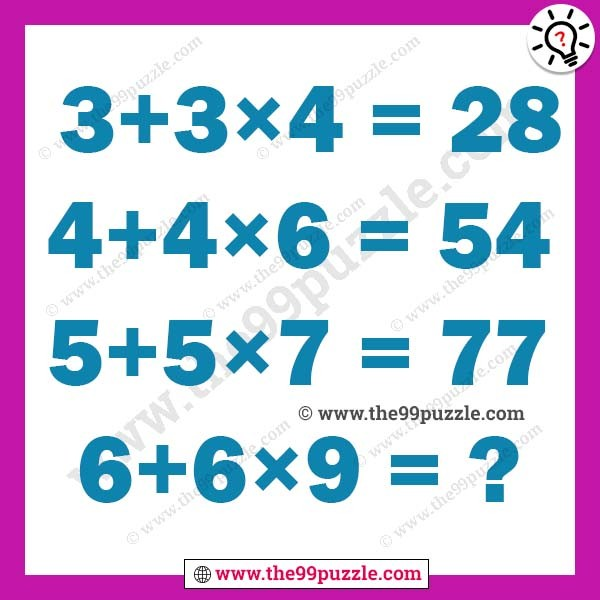5. Simple Math Logic Equation with Answer

1+5=12, 2+10=24, 3+15=36, 5+25=?6. Math equation puzzle with solution

3+5+6=151872, 5+5+6=253094, 5+6+7=303585, 5+5+3=251573, 9+4+7=?7. Math tricky riddle with answer

1+5=18, 2+7=27, 3+9=36, 5+10=45, 4+20=?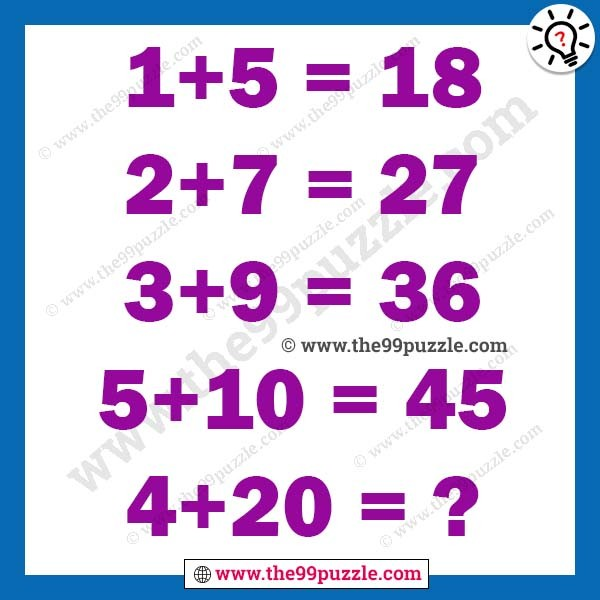8. Logical Math Equation with Answer for Teens

5+7=42, 6+8=54, 4+9=41, 7+8=60, 9+8=?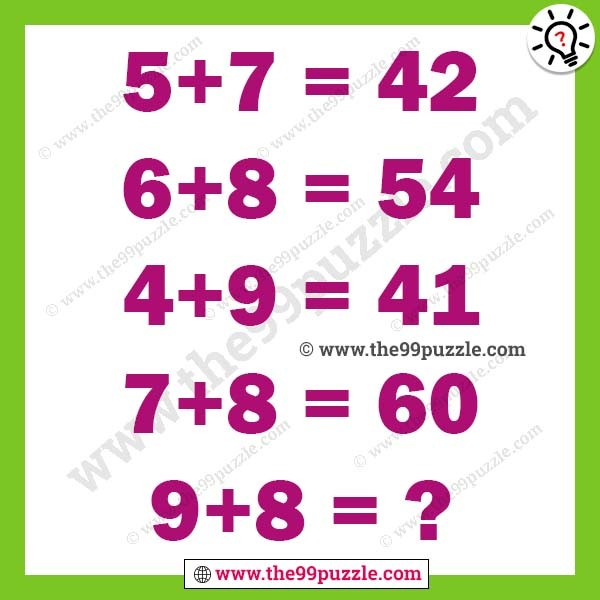9. Which number can replace the question mark in this box puzzle?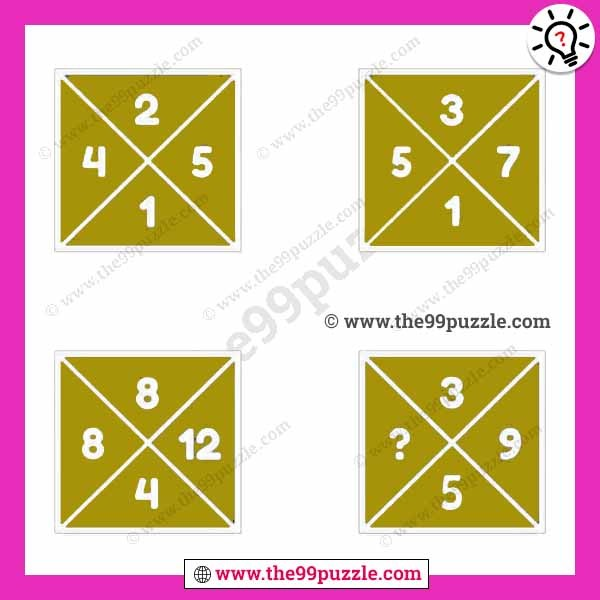10. Interesting Maths Equation Puzzle For Teens With Answer

1+1=121, 1+2=134, 2+2=444, 3+2=954, 3+3=?Here you will find many challanging puzzles, riddles, brain games, brain teases and mnay more. All these tricky math puzzles can help to exercise your brain. Get more best challenging math puzzles for genius with answer and solution.

error: